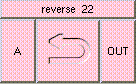REVERSETHE BOX'S MAIN HELP: Result is 1-A. This reverses the direction of A. Inputs should lie in the range [0,1]. Vectors should be normalized. A (input): The value to be reversed. The input may be a scalar, vector, or color. Inputs should lie in the range [0,1]. Vectors should be normalized. OUT (output): Result is 1-A. This reverses the direction of A. Inputs should lie in the range [0,1]. Vectors should be normalized.

<-- BACK TO Math